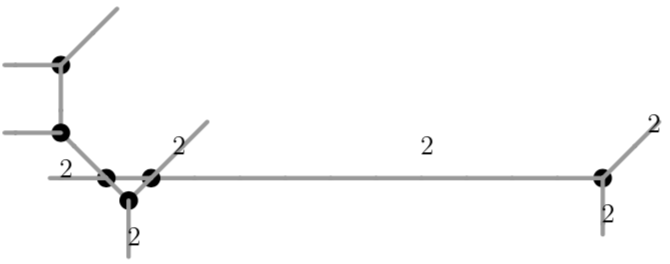# Type (111)¶

## Plane quartics of type (111)¶

A tropical curve of type (111) is of the form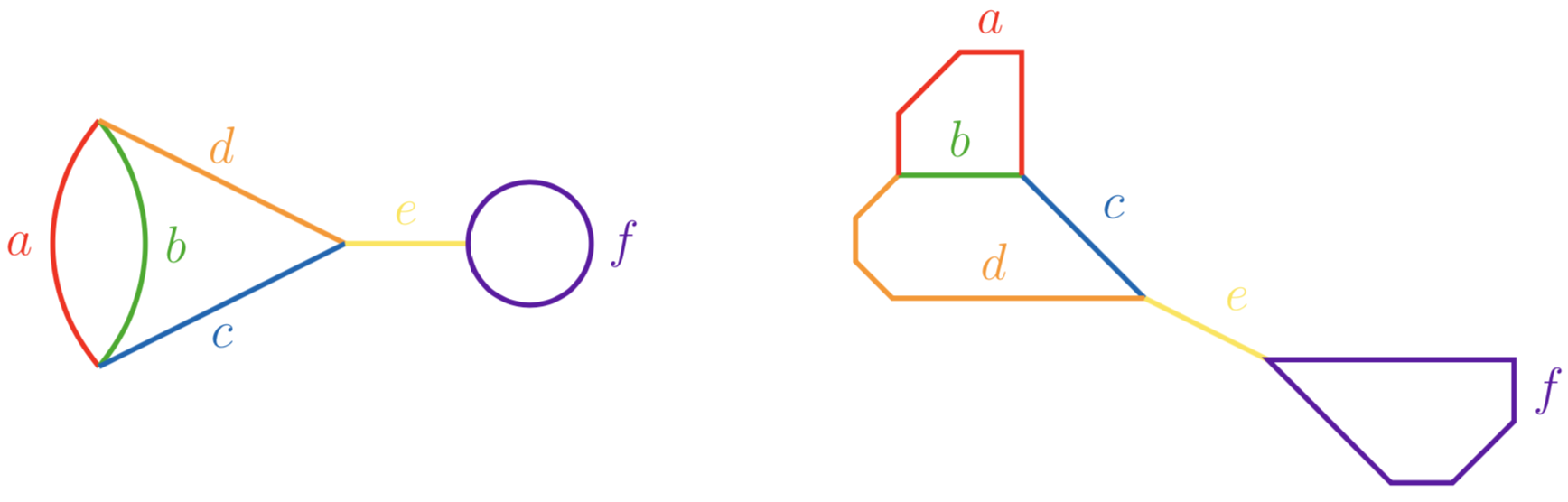By symmetry, we may assume that $$a\leq b$$ or $$b\leq a$$ and $$c\leq d$$ or $$c\geq d$$. Due to the positioning of the edges in plane tropical curves of type (111), we choose $$b\leq a$$ and $$c\leq d$$. Moreover, as the curve is not realizably hyperelliptic, we may also assume $$c\neq d$$.

These curves can be realized as tropical plane quartics if and only if $$b+c<d<b+3c$$. Hence there are two classes of tropical quartics of type (111) which cannot be realized as tropical plane quartics:

• curves with $$d\leq b+c$$

• curves with $$d\geq b+3c$$

## Quartics of type (111) with $$d\geq b+c$$¶

To realize curves with $$d\leq b+c$$, say

$a=11, \quad b=4, \quad c=3, \quad d=7, \quad e=1, \quad f=18,$

we begin with a plane tropical quartic with correct lengths $$a,c,d,e,f$$ and length $$b':=d-c$$, whose edge of length $$b'$$ we may unfold to an edge of length $$b$$. For example, the tropical plane quartic

poly g = 2t2+t3x-y+t14x2-xy+y2+t27x3+t6x2y+xy2+t4y3+t44x4+t21x3y+t2x2y2+t5xy3+t10y4;
drawTropicalCurve(g,"max");

$\begin{split}g &= 2t^2+t^3x-y+t^{14}x^2-xy+y^2+t^{27}x^3+t^6x^2y+xy^2+t^4y^3 \\ & \quad +t^{44}x^4+t^{21}x^3y+t^2x^2y^2+t^5xy^3+t^{10}y^4\end{split}$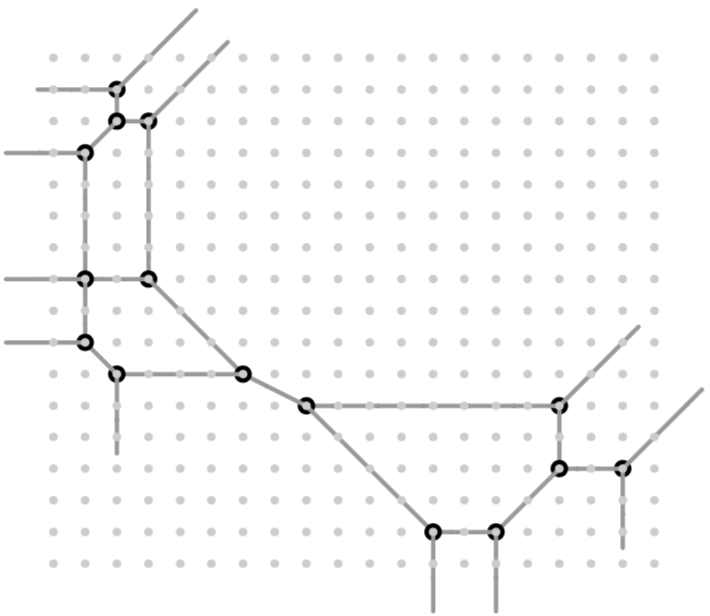reveals the actual length of edge $$b$$ to be 4 after the modification $$y=z+1$$ ($$y$$ remaining the vertical direction):

poly g1=substitute(g,y,y+1);
drawTropicalCurve(g1,"max");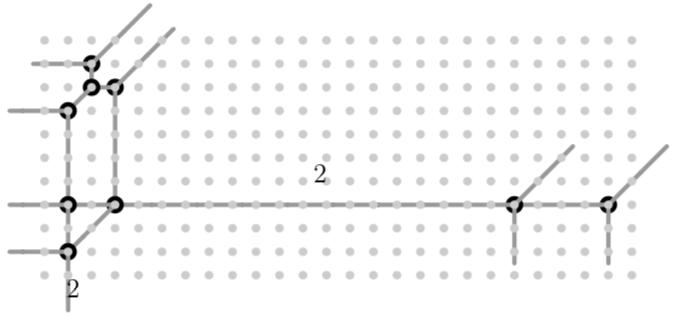Thus, the tropicalization of $$\langle g,y-(z+1) \rangle$$ yields a tropical quartic inside the tropical plane given by $$y-(z+1)$$ with the desired skeleton: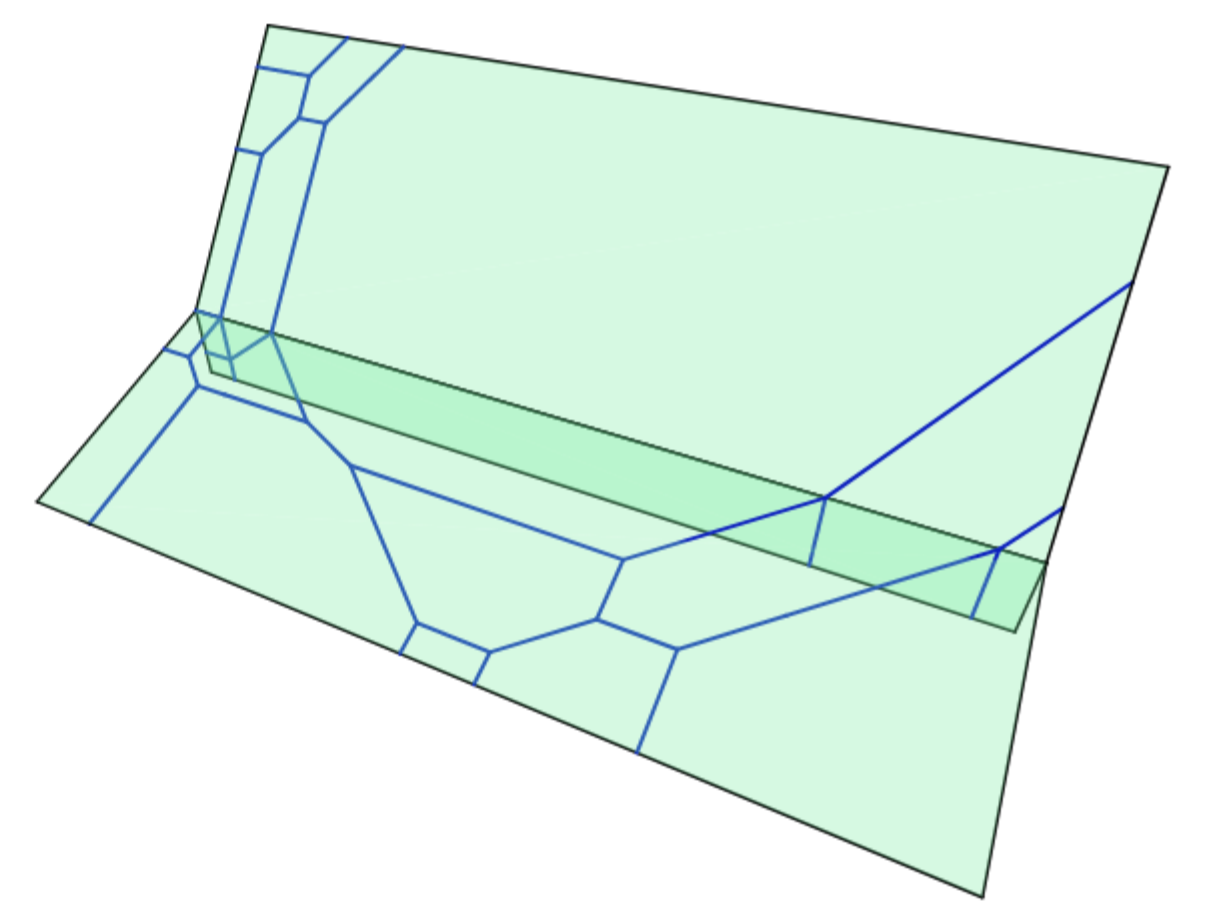## Quartics of type (111) with $$d\geq b+3c$$ and $$a<b$$¶

To realize curves with $$d>b+3c$$ and $$a>b$$, say

$a=5, \quad b=2, \quad c=3, \quad d=20, \quad e=2, \quad f=12,$

we begin with a plane tropical quartic with correct lengths $$a,b,c,d$$ and edges with higher multiplicity which reveal a bounded edge of length $$e$$ and cycle of length $$f$$ after suitable modifications. For example the tropical plane quartic

poly g = t18+t6*x+(1-t4)*x2+t24*x4+t11*y+xy-2x2y+t8*y2+t3*xy2-x2y2+t12*y3+t8*xy3+t18*y4;
drawTropicalCurve(g,"max");

$\begin{split}g &= t^{18} + t^{6}x + (1-t^4) x^2 + t^{24} x^4 + t^{11}y + xy -2 x^2y \\ &\quad + t^{8}y^2 + t^{3} xy^2 - x^2y^2 + t^{12}y^3 + t^{8}xy^3 + t^{18}y^4\end{split}$reveals an edge of length 2 and cycle of length 12 after the modification $$y=z+1+t^2$$:

poly g1=substitute(g,y,y+1+t2);
drawTropicalCurve(g1,"max");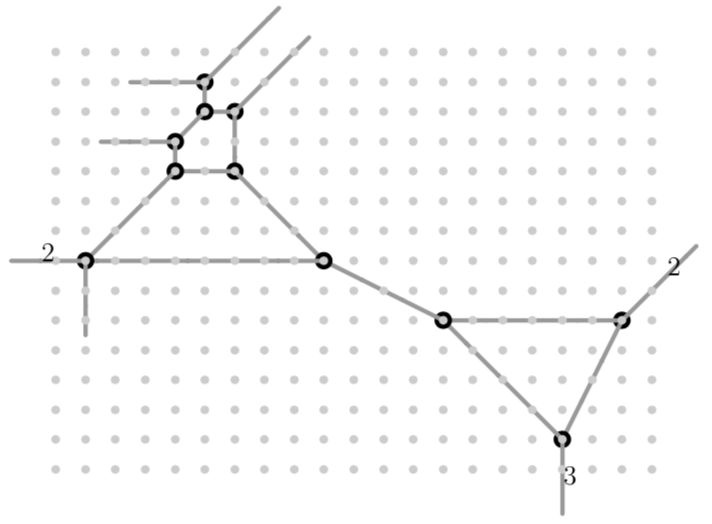Therefore, the tropicalization of $$\langle g,y-(z+1+t^2) \rangle$$ yields a tropical quartic inside the tropical plane given by $$y-(z+1+t^2)$$ with the desired skeleton: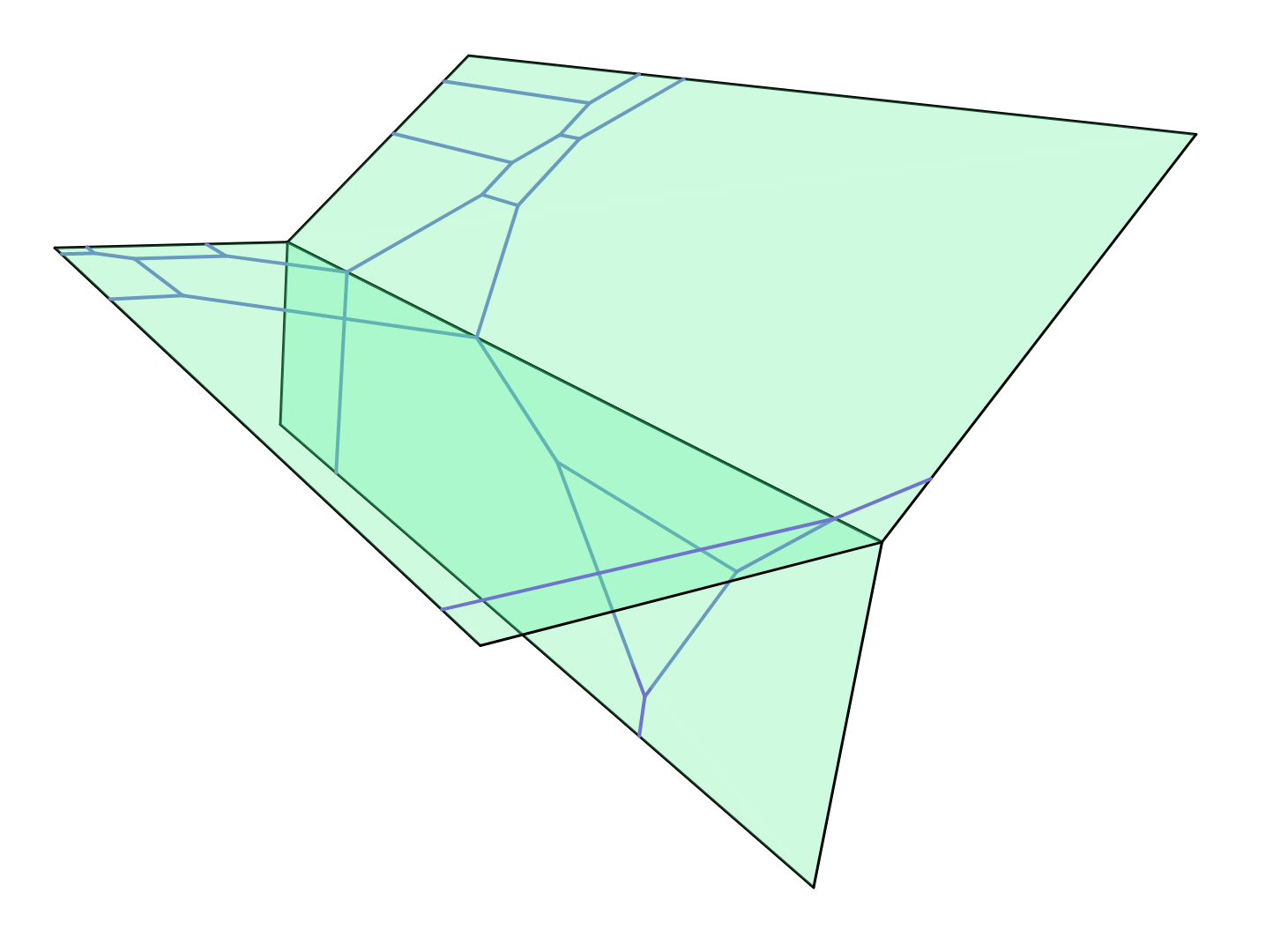## Quartics of type (111) with $$d\geq b+3c$$ and $$a=b$$¶

To realize curves with $$d>b+3c$$ and $$a=b$$, say

$a=2, \quad b=2, \quad c=3, \quad d=20, \quad e=2, \quad f=12,$

we begin with a more degenerate version of the plane starting curve in the case $$a>b$$, in which edges $$a$$ and $$b$$ are collapsed into a single edge of length $$a=b$$ of multiplicity two:

poly g = t18+t6*x+(1-t4)*x2+t24*x4+t11*y-xy-2x2y+t10*y2-x2y2+t16*y3+t8*xy3+t25*y4;
drawTropicalCurve(g,"max");

$\begin{split}g &= t^{18} + t^{6}x + (1-t^4) x^2 + t^{24} x^4 + t^{11}y + xy -2 x^2y \\ &\quad + t^{8}y^2 + t^{3} xy^2 - x^2y^2 + t^{12}y^3 + t^{8}xy^3 + t^{18}y^4\end{split}$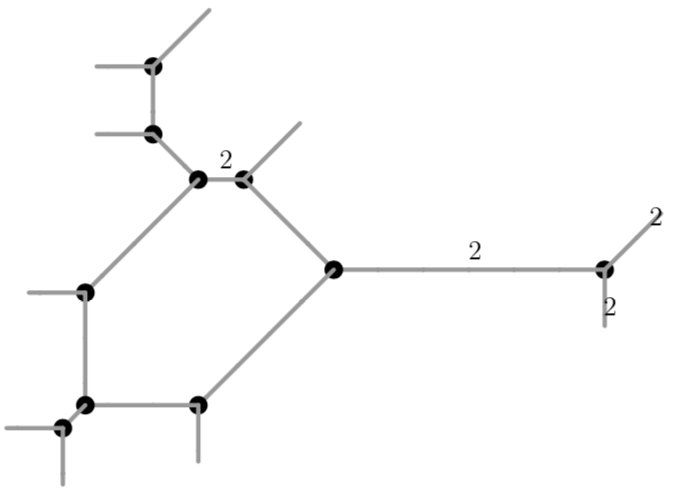A modification then seperates the two edges of equal length:

poly g1=substitute(g,y,t^(-4)*y+t^(-4));
drawTropicalCurve(g1,"max");